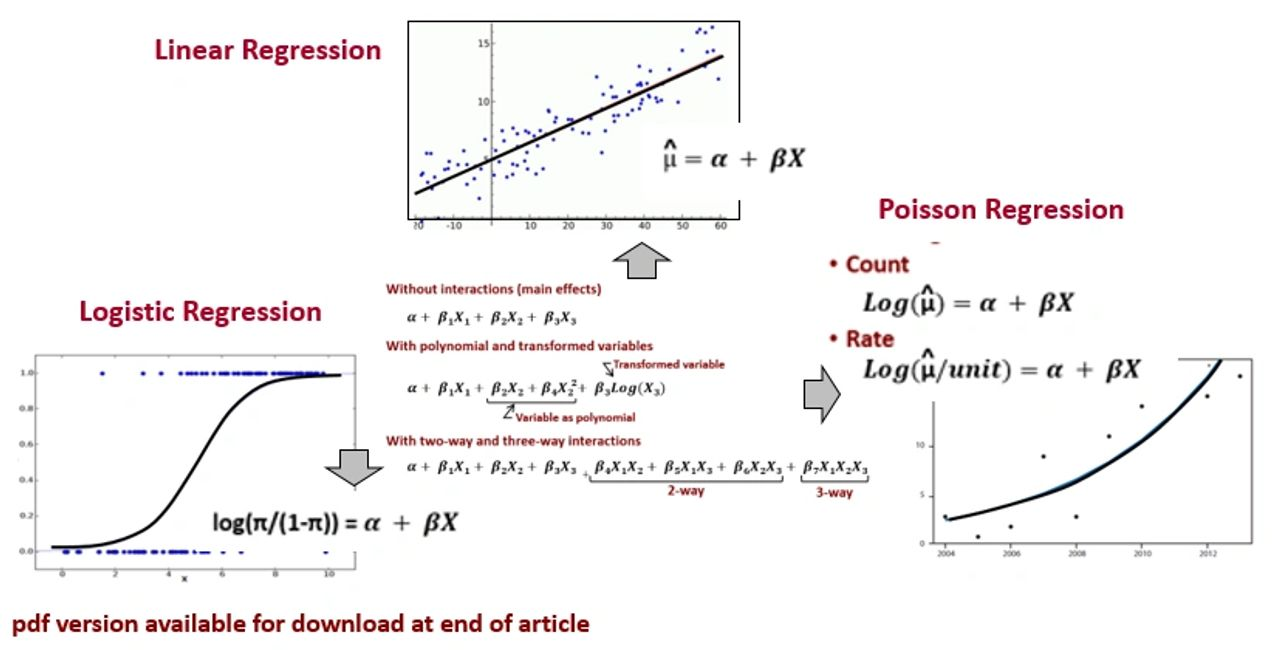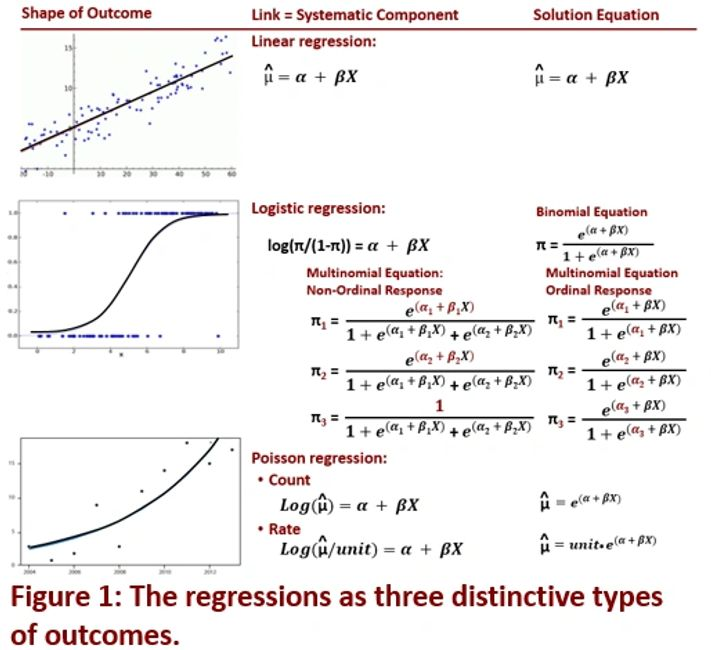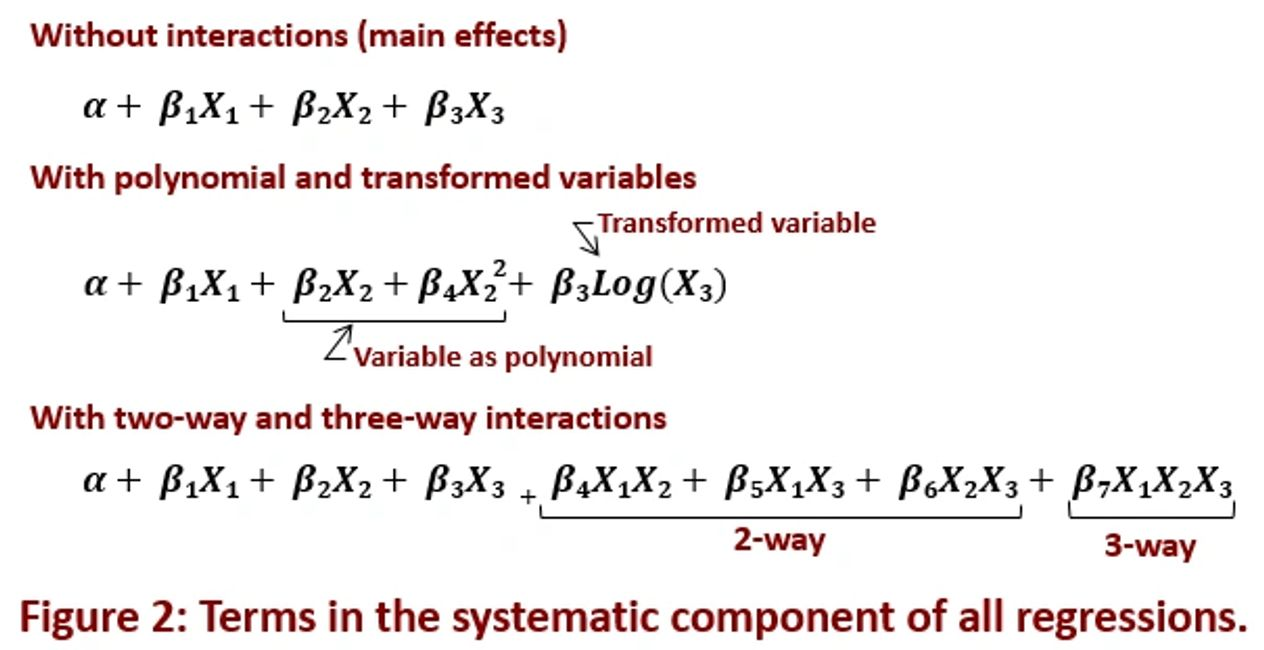New-Age Five Questions >> Find What Matters by Asking Relationship Questions of OperationsBy Richard G. Lamb, PE, CPA; Analytics4Strategy.com

The power of relationship questioning through regression models is to explore which and how strongly elements across the system of operational processes are related to outcomes and to each other. From the gained insight, firms are able to target the elements along its operations for which surgical change, improvement and assured compliance will be felt as earnings and return on investment.

This article explains relationship questioning that improvement teams must know to ask and answer of processes and the linear, logistic and Poisson regression models to do so. Relationship questioning is one of five core types of questioning—relationship, difference, time series, duration and apparency—all which play together to augment a team’s ability to reach for operational excellence.

However, the explanation we all want in the end is how to conduct the explained exploration. The article, “DMAIC Done the New-Age Way,” explains how the relationship questioning of this article, along with the four other core types of questioning are woven into the stages to define, measure, analyze, improve and control processes. Although presented in the context of DMAIC, the explanation is universal to any problem solving sequence.

Nature of Questioning

Relationship-type questions are explored with the many variations of regression models. We seek to know which and how strongly variables across the system of operational processes are related to outcomes along the system and each other.

In the context of continuous improvement, the purpose of regression models departs from conventional thinking. Most people think of regressions as models with which to predict outcomes.

In contrast, continuous improvement teams should regard the models as the as-is of the subject operational processes. We work backward from the as-is to unearth and explore the variables across the enterprise that are related to it. Upon recognizing and quantifying the relationships the team determines the longer-term can-be and then the shorter-term to-be.

Rather than the final “box” of the subject processes, the as-is, can-be and to-be of interest to the team are at outcome variables throughout a system of operational processes. We question in two directions. Which enterprise-level outcomes are the “local” outcome variables of the process related to? Which variables across the enterprise are related to the outcome variables along the processes and each other?

Three Types of Outcomes

The first question for the continuous improvement team is what type of outcome is being measured at each outcome variable? The question goes unasked because most of us think in terms of a number is a number.

There are actually three types of outcomes. First are continuous numeric outcomes such as temperature, cost and productivity. Second are the proportion of times something happens to fall into one of two or more classifications. Third are counts and rates.

In statistics-speak, they are linear (continuous numeric), logistic (proportions) and Poisson (counts and rates). Figure 1 shows the difference between them with respect to shape and their contrasting characteristics.

As mine does, I’m sure your heart always sinks at the first glance of something like Figure 1. However, the take-away is simple. Life will be good if we do not confuse the type of outcome we are modeling.

The purpose of the figure is to give you the big picture. The big picture is that all models entail a linking function, systematic component and solution equation. All will be appropriately handled by the software once we correctly tell it which type of outcome we are working with.

The first thing to notice is that the “systematic component” (α + βX) of the three regressions is the same. The distinction is how the systematic component is mathematically translated to an answer. The translation is according to a “linking function” as shown on the left side of the equality symbol. Because of the arithmetic of linking functions, the coefficient (β) tells a different story for each type of outcome.The first regression is conveniently called “linear” because its results will always fall along a straight line. The outcome can range from positive to negative infinity, whereas, the others obviously cannot.

The linking function is called the “identity.” This is because what the systematic component generates is identical to what we get. No further arithmetic takes place. The coefficient times one unit or level of change of the associated variable (X) adds or subtracts from the outcome.

It is safe to say that linear models probably depict much less of operational processes than do the other two regressions. This is because most operational processes predominately entail classifications, counts and rates rather than continuous numeric readouts.

The second case of the figure, logistic regression, is the “regression of classifications.” It deals with binary outcomes (yes, no) and multinomial outcomes. Multinomial outcomes can be nominal (red, white, blue) or ordinal (low, medium, high).

Logistic regressions relate process variables to “odds” (proportion of yes divided by the proportion of no; π/(1- π)) of a particular outcome versus another. Another interpretation of logistic regression is that it speaks to the likelihood of a particular classification given different situations.

The output is the expected proportion (π) of a binomial or multinomial outcome. Accordingly, the outcome (π) always falls between zero and one rather than positive or negative infinity.

Because the identity link does not apply, the result (proportion) for each setting of the systematic component is determined by the calculation shown in Figure 1. Although it looks different, the binomial equation is actually a special case of the nominal multinomial equation.

The exponential (e) of the coefficient reflects how the odds ratios (odds of one setting of the systematic body divided by the odds of an alternative setting) will be multiplied by one unit or level of change to a related variable.

The third case of Figure 1, Poisson regression, is concerned with counts and rates. “Rates” tie counts to time, space or some other index of size (e.g., events per 1,000 hours).

The linking function is the log of the mean (log linear; log(µ)). Therefore, its outcome can only be zero or greater. The coefficients have the same exponentiated effect on the counts and rates as in logistic regression. Counts and rates are determined by the applicable calculations shown in Figure 1.

What to Leave In, What to Leave Out

Now the goal is to identify the one or more variables that are related to the outcome variables of interest. Is there a relationship? Is there a relationship, but somehow distorted? Is the relationship significant? What is the direction of the relationship? Are the variables related to each other? Are any of the variables related to others that are not included in the model?

The measure of how well a model meets the goal is how much of the variance around the overall average, proportion, count or rate of the data is explained by the included variables and terms. Too few and much of the variance is left unexplained. Too many and it is difficult to interpret the findings.

The questions of relationship boil down to an initial overarching question to be answered in iteration. What to leave in; what to leave out?

The variables in a model are variously structured as terms as shown in Figure 2. In the upper systematic component, the three variables (X1, X2 and X3) are included as single terms (called main effects). In the middle systematic component, one variable is included as terms of a polynomial (X2) and one as a transformation (X3). In the bottom systematic component, all three variables are included as main effects and as orders of interactions.

Once again, stay calm. We are not taking the watch apart to explain how to tell time. The simple takeaway of the figure is that the systematic component will ultimately be a set of terms. The set can range from only one main effect to engaging some or all of the main effects in multiple terms that best tell the story. The variables in the model can also be numeric and categorical.The in-or-out question is answered as the model is iteratively built and run until the team converges on the model that best depicts all of the relationships that will be significant to improving the subject operational process. All along, the expertise of the people who know the process and enterprise will influence the choices.

The expertise-driven iterations to evaluate the significance of each term begins with eliminating any insignificant interaction terms; from the highest order downward. Thence, the significance of the main effects (if not included in a significant interactive term) and terms are evaluated for pruning. Somewhere in the iterations the team may find it necessary to try transformations and polynomial effects.

The team has found its variables when it reaches its final iteration. But wait, there is another question to ask of the selected variables. Are any of the variables related to others across the system of processes, but are not included in the model? If the question is not asked, the team could build solutions around a seeming relationship that is actually reflecting another.

The iterative winnowing process is done real-time in a team meeting. The continuous improvement leader facilitates the model-directed discussion until the team settles on one that best tells the story. There will be discovery along the way for the subject matter experts and operatives; to quote Johnny Carson, “I did not know that!”

Hunt Down the Trolls

Statistics do not lie; people lie with statistics. Truthful models come out of being subjected to considerable validating diagnostics. This article will not go into them as they are a big topic in their own right.

Now that the team has a well-fitting model it still must confirm that it is a truthful model. Now the team will get the dogs out and hunt for trolls. The search will be through both variables and individual cases; methodically asking a range of diagnostic questions.

With respect to variables, the questions will test independence, consistent variance of residuals, normality of outcome residuals and collinearity.

With respect to cases, the questioning will seek outliers to the model, as well as, excessive influences on it.

When spotted, the team will want to understand how the trolls are able to thrive. The result will be discovery, cleansing and transformations. It may also beget immediate changes to the subject operational process and others.

The questioning process to find the trolls is a real-time team discussion led by the continuous improvement leader. Depending upon the findings, the discussion may return to the iterative leave-in, leave-out discussion. If so, the thrust will be to modify the model’s systematic component.

Question the Hierarchy

By “question the hierarchy” we are not talking about “stick it to the man.” The team has settled on the relationships that matter and eliminated any hiding trolls. However, the team should not yet assume that the model realistically depicts the situation.

There is a final question. Which, if any, of the relationships are hierarchical?

As the explanation of hierarchy unfolds, it will be apparent that it is an extremely important question. This is because multiple-level structure is typical to enterprise operations.

The concept of level is a simple, but entails a mind-boggling technical explanation. Fortunately, an example of a simple linear regression model with one input variable will make it clear. The difference is level.

Imagine a model built to relate the day’s workload to productivity—hours per task. However, is it truthful to model productivity across the entire enterprise as if a single level? In other words, are the intercept and coefficient (β) consistent across the enterprise? Or, do they vary by department? Answering the question requires that the team builds what is called a “multiple level model.”

Figure 3 shows the idea with respect to a linear regression. Look at it and think simply. Also make note that the method plays for logistic and Poisson regressions.The left side of Figure 3 shows a single-level model. This is the perspective we all grew up with. The intercept and slope between the input (systematic component) and outcome variables are the same the across the enterprise.

The right side of the figure presents another reality—two levels. Imagine three departments (level two) across the enterprise in which the same activity is conducted. The figure depicts that the three departments have different intercepts and coefficients. Each varies from the overall model that is shown on the left side of the figure.

In a nutshell, the team needs to determine if the reality of each relationship is to the left or right side of the figure. The team also needs to confirm that the variances in intercepts and coefficients from the overall model are significant.

The example is the simplest of all possibilities. The levels can go beyond two. Imagine expanding the hierarchical levels to location, department and crew. Further yet, imagine selectively attaching variables to different levels.

To establish or rule out multiple-level relationships, the team will build a multiple level model. In a team session, the model will be built up one variable, one intercept and one coefficient at a time. At each build, the team will evaluate whether or not the model fits better than the previous iteration. “Better” indicates that the currently modeled iteration is the actual case.

Sources for self-directed learning: Discovering Statistics Using R, Field and Miles, 2012 | Multilevel Modeling Using R, Holmes, 2014 | Machine Learning with R, Lantz, 2015 | ggplot2, Elegant Graphics for Data Analysis, Wickham, 2016 | Introductory Time Series with R, Cowpertwait and Metcalfe, 2009 | Event History Analytics with R, Bostrom, 2012 | Package “tsoutliers,” Javier López-de-Lacalle, 2017